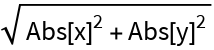# RealEuclideanDistance

Contributed by: Ed Pegg Jr

gives the Euclidean distance between two real vectors without using absolute value

 ResourceFunction["RealEuclideanDistance"][u,v] gives the Euclidean distance between real vectors u and v without using Abs[].

## Examples

### Basic Examples

Compute the real Euclidean distance between two points:

 In:=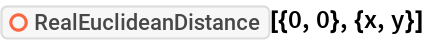Out=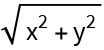Compute in three dimensions:

 In:=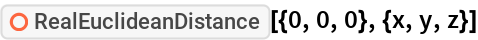Out=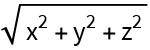Using numeric values:

 In:=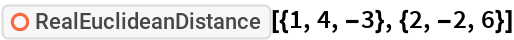Out=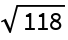### Properties and Relations

EuclideanDistance adds Abs for variables and complicated expressions, making them more complicated. Often, that extra complication will make Simplify more difficult:

 In:=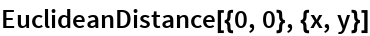Out=